# Selina solutions for Concise Physics Class 10 ICSE chapter 7 - Sound [Latest edition]

#### Chapters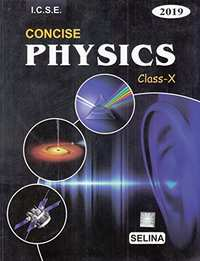## Chapter 7: Sound

Exercise 7 (A)Exercise 7 (B)Exercise 7 (C)
Exercise 7 (A) [Page 154]

### Selina solutions for Concise Physics Class 10 ICSE Chapter 7 Sound Exercise 7 (A) [Page 154]

Exercise 7 (A) | Q 1 | Page 154

What are mechanical waves?

Exercise 7 (A) | Q 2.1 | Page 154

Define amplitude term in relation to a wave.

Exercise 7 (A) | Q 2.2 | Page 154

Define frequency term in relation to a wave.

Exercise 7 (A) | Q 2.3 | Page 154

Define wavelength term in relation to a wave.

Exercise 7 (A) | Q 2.4 | Page 154

Define wave velocity term in relation to a wave.

Exercise 7 (A) | Q 3.1 | Page 154

A wave passes from one medium to another medium. Mention the property of the wave which changes.

Exercise 7 (A) | Q 3.2 | Page 154

A wave passes from one medium to another medium. Mention the property of the wave which does not change.

Exercise 7 (A) | Q 4 | Page 154

State two factors on which the speed of a wave travelling in a medium depends.

Exercise 7 (A) | Q 5 | Page 154

State two differences between the light and sound waves.

Exercise 7 (A) | Q 6 | Page 154

What do you mean by reflection of sound? State one condition for the reflecton of a sound wave. Name a device in which reflection of sound wave is used.

Exercise 7 (A) | Q 7.1 | Page 154

What is meant by an echo?

Exercise 7 (A) | Q 7.2 | Page 154

What is the condition necessary for an echo to be heard distinctly?

Exercise 7 (A) | Q 8 | Page 154

A man is standing at a distance of 12 m from a cliff. Will he be able to hear a clear echo? Give a reason for your answer.

Exercise 7 (A) | Q 9 | Page 154

State two applications of echo.

Exercise 7 (A) | Q 10 | Page 154

Explain how the speed of sound can be determined by the method of echo.

Exercise 7 (A) | Q 11 | Page 154

State the use of echo by a bat, dolphin and fisherman.

Exercise 7 (A) | Q 12 | Page 154

How do bats avoid obstacles in their way, when in flight?

Exercise 7 (A) | Q 13.1 | Page 154

What is meant by sound ranging?

Exercise 7 (A) | Q 13.2 | Page 154

Give one use of sound ranging.

Exercise 7 (A) | Q 14 | Page 154

Name the waves used for sound ranging. Why are the waves mentioned by you audible to us?

Exercise 7 (A) | Q 15 | Page 154

What is sonar? State the principle on which it is based.

Exercise 7 (A) | Q 16 | Page 154

State the use of echo in medical science.

Exercise 7 (A) [Page 154]

### Selina solutions for Concise Physics Class 10 ICSE Chapter 7 Sound Exercise 7 (A) [Page 154]

Exercise 7 (A) | Q 1 | Page 154

The minimum distance between the source and the reflector in air. So that an echo is heard is approximately equal to:

• 10 m

• 17 m

• 34 m

• 50 m

Exercise 7 (A) | Q 2 | Page 154

To detect the obstacles in their path, bats produce:

• Infrasonic waves

• Ultrasonic waves

• Electromagnetic waves

Exercise 7 (A) [Pages 154 - 155]

### Selina solutions for Concise Physics Class 10 ICSE Chapter 7 Sound Exercise 7 (A) [Pages 154 - 155]

Exercise 7 (A) | Q 1 | Page 154

The wavelength of waves produces on the surface of water is 20 cm. If the wave velocity is 24 m s-1, calculate: (i) the number of waves produces in one second , and (ii) the time in which one wave is produced.

Exercise 7 (A) | Q 2 | Page 154

Calculate the minimum distance in air required between the source of sound and the obstacle to hear an echo. Take speed of sound in air = 350 m s-1

Exercise 7 (A) | Q 3 | Page 155

What should be the minimum distance between source and reflector in water so that echo is heard distinctly?
(The speed of sound in water = 1400 m s-1)

Exercise 7 (A) | Q 4 | Page 155

A man standing 25 m away from a wall produces a sound and receives the reflected sound. (a) Calculate the time after which he receives the reflected sound if the speed of the sound in air is 350 m s-1. (b) will the man be able to hear a distinct echo? Explain the answer.

Exercise 7 (A) | Q 5 | Page 155

A radar sends a signal to an aeroplane at a distance 45 km away with a speed of 3 × 108 m s -1. After how much time is the signal received back from the aeroplane?

Exercise 7 (A) | Q 6 | Page 155

A man standing 48 m away from a wall fires a gun calculate the time after which an echo is heard. (The speed of sound in air is 320 m s -1).

Exercise 7 (A) | Q 7 | Page 155

A ship on the surface of water sends a signal and receives it back froma a submarine inside water after 4 s. Calculate the distance of the submarine from the ship. (The speed of sound in water is 1450 m s-1)

Exercise 7 (A) | Q 8 | Page 155

A pendulum has a frequency of 5 vibrations per second. An observer starts the pendulum and fires a gun simultaneously. He hears echo from the cliff after 8 vibrations of the pendulum. If the velocity of sound in air is 340 m s-1, find the distance between the cliff and the observer.

Exercise 7 (A) | Q 9 | Page 155

A person standing between the two vertical cliffs produces a sound. Two successive echoes are heard at 4 s and 6 s. Calculate the distance between the cliffs.
(speed of sound in air = 320 m s-1)

Exercise 7 (A) | Q 10 | Page 155

A person standing at a distance x in front of a cliff fires a gun. Another person B standing behind the person A at a distance y from the cliff hears two sounds of the fired shots after 2s and 3s respectively. Calculate x and y(take speed of sound 320m/s).

Exercise 7 (A) | Q 11 | Page 155

On sending an ultrasonic wave from a ship towards the bottom of a sea, the time interval between sending the wave and receiving it back is found to be 1.5 s. If the velocity of wave in sea water is 1400 m s-1, find the depth of the sea.

Exercise 7 (A) | Q 12 | Page 155

Figure below shows the distance-displacement graph of two waves A and B. Compare (i) the amplitude, (ii) the wavelength of the two waves.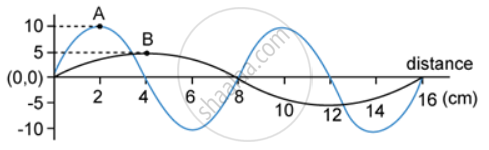Exercise 7 (B) [Pages 163 - 165]

### Selina solutions for Concise Physics Class 10 ICSE Chapter 7 Sound Exercise 7 (B) [Pages 163 - 165]

Exercise 7 (B) | Q 1 | Page 163

What do you understand by free vibrations of a body?

Exercise 7 (B) | Q 2.1 | Page 163

What is meant by the natural frequency of vibration of a body?

Exercise 7 (B) | Q 2.2 | Page 163

On What factors does the natural frequency of vibration of a body depend?

Exercise 7 (B) | Q 3 | Page 163

Draw a graph between displacement from mean position and time for a body executing free vibrations in vacuum.

Exercise 7 (B) | Q 4 | Page 163

State one condition for a body to execute free vibrations.

Exercise 7 (B) | Q 5 | Page 163

(a) Name one factors on which the frequency of sound emitted due to vibrations in an air column depends.

(b) How does the frequency depend on the factor stated in part (a).

Exercise 7 (B) | Q 6 | Page 164

State one way of increasing the frequency of a note produced by an air column.

Exercise 7 (B) | Q 7 | Page 164

State two ways of increasing the frequency of vibrations of a stretched string.

Exercise 7 (B) | Q 8 | Page 164

How does the frequency of sound given by a stretched string depend on its (a) length, (b) tension?

Exercise 7 (B) | Q 9 | Page 164

What adjustments would you make for tuning a stringed instrument for it to emit a note of a desired frequency?

Exercise 7 (B) | Q 10 | Page 164

The following diagram shows three ways in which the string of an instrument can vibrate.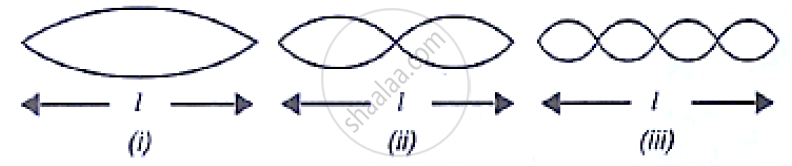(a) which of the diagram shows the principal note?
(b) which has the frequency four times that of the first?
(c) what is the ratio of the frequency of the vibration in (i) and (ii)?

Exercise 7 (B) | Q 11 | Page 164

Explain why strings of different thicknesses are provided on a stringed instrument.
[Hint : Natural frequency of vibration of a stretched string is inversely proportional to the radius (or thickness) of string so notes of different frequencies can be produces by vibrating different strings.]

Exercise 7 (B) | Q 12 | Page 164

A blade, fixed at one end, is made to vibrate by pressing its other end and then releasing it. State one way in which the frequency of vibrations of the blade can be lowered.

Exercise 7 (B) | Q 13 | Page 164

How does the medium affect the amplitude of free vibrations of a body?

Exercise 7 (B) | Q 14 | Page 164

What are damped vibrations? How do they differ from free vibrations? Give one example of each.

Exercise 7 (B) | Q 15 | Page 164

The following diagram shows the displacement – time graph of the vibrating body.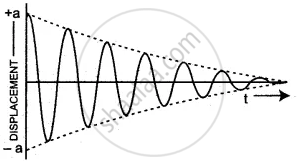(i) name the kind of vibrations
(ii) Give one example of such vibrations
(iii) why is the amplitude of vibrations gradually decreasing?
(iv) what happens to the vibrations of the body after some time?

Exercise 7 (B) | Q 16 | Page 164

A tuning fork is set into vibration in air. Name the kind of vibrations it executes.

Exercise 7 (B) | Q 17 | Page 164

Draw a sketch showing the displacement of a body executing damped vibrations against time.

Exercise 7 (B) | Q 18.1 | Page 164

What are forced vibrations?

Exercise 7 (B) | Q 18.2 | Page 164

Give one example to illustrate forced vibrations.

Exercise 7 (B) | Q 19 | Page 164

On keeping the stem of a vibrating tuning fork on the surface of a table, a loud sound is heard. Give reason.

Exercise 7 (B) | Q 20 | Page 164

Distinguish between the free (or natural) and forced vibrations.

Exercise 7 (B) | Q 21.1 | Page 164

What is meant by resonance?

Exercise 7 (B) | Q 21.2 | Page 164

Describe a simple experiment to illustrate the phenomenon of resonance and explain it.

Exercise 7 (B) | Q 22 | Page 164

State the condition for the occurrence of resonance.

Exercise 7 (B) | Q 23 | Page 164

Complete the following sentence:
Resonance is a special case of …………….. vibrations, when frequency of the driving force is ……… natural frequency of the body.

Exercise 7 (B) | Q 24 | Page 164

Differentiate between the forced and resonant vibrations.

Exercise 7 (B) | Q 25 | Page 164

Why is a loud sound heard at resonance?

Exercise 7 (B) | Q 26 | Page 164

In following figure shows two tuning forks A and B of the same frequency mounted on separate sound boxes with their open ends facing each other. The fork A is set into vibration. (a) Describe your observation. (b) state the principle illustrated by this experiment.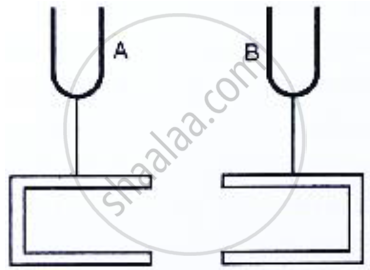Exercise 7 (B) | Q 27 | Page 165

In following figure shows  A, B, C and D are four pendulums suspended from the same elastic string XY. Lengths of pendulum A and D are equal, while the length of pendulum B is smaller and the pendulum C is longer. The pendulum A is set into vibration.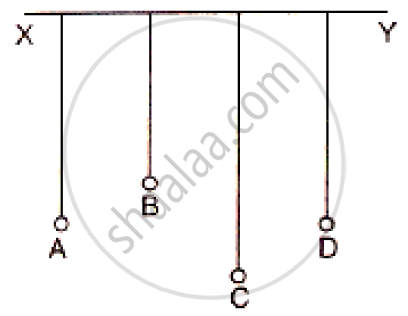Exercise 7 (B) | Q 28 | Page 165

A vibrating tuning fork held over an air column of a given length with its one end closed, produces a loud audible sound. Name the phenomenon responsible for it and explain the observation.

Exercise 7 (B) | Q 29 | Page 165

In following figure shows  A, B , C and D represent test tube each of height 20 cm which are filled with water up to heights of 12 cm, 14 cm, 16cm and 18cm respectively. If a vibrating tuning fork is placed over the mouth if test tube D, a loud sound is heard.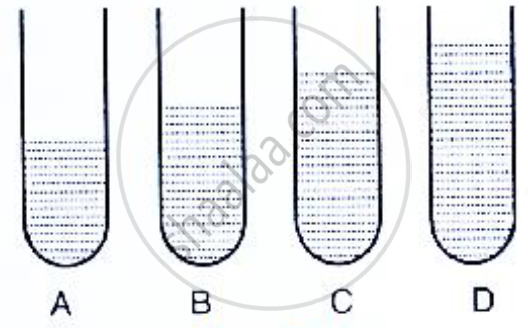(a) Describe the observations with the tubes A, B and C when the vibrating tuning fork is placed over the mouth of these tubes.
(b) Give the reason for your observation in each case.
(c) State the principle illustrated by the above experiment.

Exercise 7 (B) | Q 30 | Page 165

When a troop crosses a suspension bridge the soldiers are asked to break steps. Explain the reason.

Exercise 7 (B) | Q 31 | Page 165

Why are the stringed instruments like guitar provided with a hollow sound box?

Exercise 7 (B) | Q 32.1 | Page 165

Exercise 7 (B) | Q 32.2 | Page 165

Name the phenomenon involved in tuning your radio set to a particular station  and define it.

Exercise 7 (B) [Page 165]

### Selina solutions for Concise Physics Class 10 ICSE Chapter 7 Sound Exercise 7 (B) [Page 165]

Exercise 7 (B) | Q 1 | Page 165

A wire stretched between two fixed supports, is plucked exactly in the middle and then released. It executes (neglect the resistance of the medium):

• Resonant vibrations

• natural vibrations

• Damped vibrations

• Forced vibrations

Exercise 7 (B) | Q 2 | Page 165

When a body vibrates under a periodic force, the vibrations of the body are:

• natural vibrations

• Damped vibrations

• Forced vibration

• Resonant vibrations

Exercise 7 (B) | Q 3 | Page 165

A tuning fork of frequency 256 Hz will resonate with another tuning fork of frequency:

• 128 Hz

• 256 Hz

• 384 Hz

• 512 Hz

Exercise 7 (C) [Pages 172 - 174]

### Selina solutions for Concise Physics Class 10 ICSE Chapter 7 Sound Exercise 7 (C) [Pages 172 - 174]

Exercise 7 (C) | Q 1 | Page 172

Name three characteristics of a musical sound.

Exercise 7 (C) | Q 2 | Page 172

(a) Which of the following quality determines the loudness of a sound wave?
(i) wavelength (ii) frequency and (iii) amplitude
(b) How is loudness related to the quantity mentioned above in part(a)?

Exercise 7 (C) | Q 3 | Page 173

If the amplitude of a wave is doubled, what will be the effect on its loudness?

Exercise 7 (C) | Q 4.1 | Page 173

Two waves of the same pitch have amplitudes in the ratio 1:3. What will be the ratio of loudness?

Exercise 7 (C) | Q 4.2 | Page 173

Two waves of the same pitch have amplitudes in the ratio 1:3. What will be the ratio of frequencies?

Exercise 7 (C) | Q 5 | Page 173

How does the wave pattern of a loud note differ from a soft note? Draw a diagram.

Exercise 7 (C) | Q 6 | Page 173

Name the unit in which loudness of sound is measured.

Exercise 7 (C) | Q 7 | Page 173

Why is the loudness of the sound heard by a plucked wire increased when it is mounted on a sound board?

Exercise 7 (C) | Q 8 | Page 173

Define the term intensity of a sound wave. State the unit in which it is measured.

Exercise 7 (C) | Q 9 | Page 173

How is loudness of sound related to the intensity of wave producing it?

Exercise 7 (C) | Q 10 | Page 173

Comment on the statement ‘loudness of sound is a subjective quantity, while intensity is an objective quantity.

Exercise 7 (C) | Q 11 | Page 173

State three factors on which loundess of sound heard by a listener depends.

Exercise 7 (C) | Q 12 | Page 173

The bells of a temple are big in size. Why?

Exercise 7 (C) | Q 13 | Page 173

Name the unit used to measure the sound level.

Exercise 7 (C) | Q 14 | Page 173

What is the safe limit of sound level in dB for our ears?

Exercise 7 (C) | Q 15.1 | Page 173

What is meant by noise pollution?

Exercise 7 (C) | Q 15.2 | Page 173

Name one source of sound causing noise pollution.

Exercise 7 (C) | Q 16 | Page 173

What determines the pitch of a sound?

Exercise 7 (C) | Q 17 | Page 173

Name the subjective property of sound related to its frequency.

Exercise 7 (C) | Q 18 | Page 173

Name and define the characteristic which enables one to distinguish two sounds of same loudness, but of different frequencies, given by the same instrument.

Exercise 7 (C) | Q 19 | Page 173

Draw a diagram to show the wave pattern of high pitch note and a low pitch note, but of the same loudness.

Exercise 7 (C) | Q 20 | Page 173

How is it possible to detect the filling of a bottle under a water tap by hearing the sound at a distance?

Exercise 7 (C) | Q 21 | Page 173

The frequencies of notes given by flute, guitar and 500 Hz. Which one of these has the highest pitch?

Exercise 7 (C) | Q 22.1 | Page 173

Complete the following sentence:
The pitch of sound increases if its frequency …………..

Exercise 7 (C) | Q 22.2 | Page 173

Complete the following sentence:
If the amplitude of a sound is halved, its loudness becomes ………………

Exercise 7 (C) | Q 23 | Page 173

The diagram below shows three different modes of vibration P, Q and R of the same string of a givens length.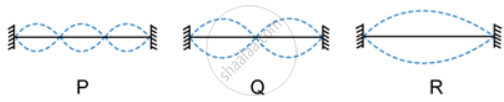(a) Which vibration will produce a louder sound and why?

(b) Which vibration will produce sound of maximum shrillness (or pitch) and why?

(c) What is the ratio of wavelength of vibrations P and R?

Exercise 7 (C) | Q 24 | Page 173

Name the characteristic which enables one to distinguish the sound of two musical instruments even if they are of the same pitch and same loudness.

Exercise 7 (C) | Q 25 | Page 173

How does the two sounds of same loudness and same pitch produced by different instruments differ? Draw diagrams to illustrate your answer.

Exercise 7 (C) | Q 26 | Page 173

Two identical guitars are played by two persons to give notes of the same pitch. Will they differ in quality? Give a reason for your answer.

Exercise 7 (C) | Q 27 | Page 173

Two musical notes of the same pitch and same loudness are played on two different instruments. Their wave patterns are as shown in following Figure.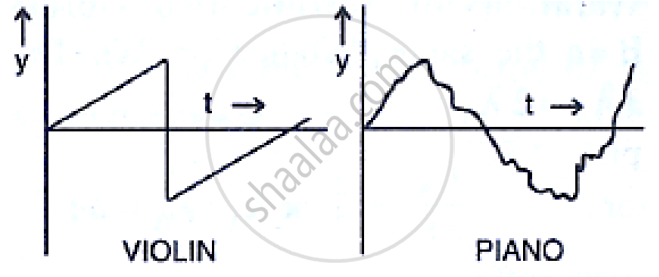Explain why the wave patterns are different.

Exercise 7 (C) | Q 28 | Page 173

How is it possible to recognize a person by his voice without seeing him?

Exercise 7 (C) | Q 29.1 | Page 173

State the factor that determine the pitch of a note.

Exercise 7 (C) | Q 29.2 | Page 173

State the factor that determine the loudness of the sound heard.

Exercise 7 (C) | Q 29.3 | Page 173

State the factor that determine the quality of the note.

Exercise 7 (C) | Q 30.1 | Page 174

Name the characteristic of the sound affected due to a change in its amplitude.

Exercise 7 (C) | Q 30.2 | Page 174

Name the characteristic of the sound affected due to a change in its wave form.

Exercise 7 (C) | Q 30.3 | Page 174

Name the characteristic of the sound affected due to a change in its frequency.

Exercise 7 (C) | Q 31 | Page 174

The sketches I to IV in following Figure show sound waves, all formed in the same time interval.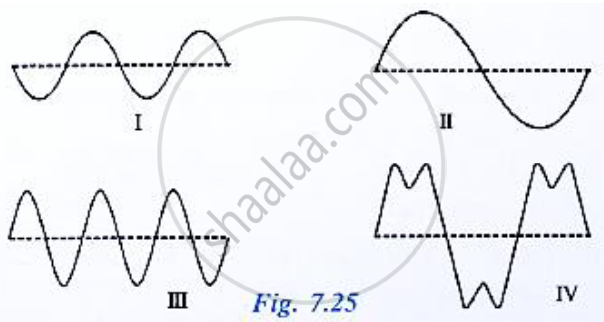Which diagram shows
(i) a note from a musical instrument
(ii) a soft (not loud) note,
(iii) a bass (low frequency) note.

Exercise 7 (C) | Q 32 | Page 174

Shows the wave patterns of three sounds A, B and C. Name the characteristic of sound which is same between (i) A and B, (ii) B and C, and (iii) C and A.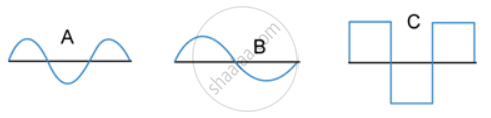Exercise 7 (C) | Q 33 | Page 174

A microphone is connected to the Y-input of a C.R.O Three different sounds are made in turn in front of the microphone. Their traces (a), (b) and (c) produces on the screen are shown in following figure .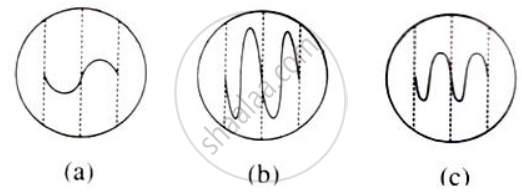(i) which trace is due to the loudest sound? Give reason for your answer.
(ii) Which trace is due for the sound with the lowest pitch? Explain your answer.

Exercise 7 (C) | Q 34 | Page 174

In what respect does the wave pattern of a noise and a music differ? Draw diagram to explain your answer.

Exercise 7 (C) | Q 35 | Page 174

State one difference between a musical note and a noise.

Exercise 7 (C) [Page 174]

### Selina solutions for Concise Physics Class 10 ICSE Chapter 7 Sound Exercise 7 (C) [Page 174]

Exercise 7 (C) | Q 1 | Page 174

By reducing the amplitude of a sound wave, its:

• Pitch increases

• Loudness decreases

• Loudness increases

• Pitch decreases

Exercise 7 (C) | Q 2 | Page 174

Two sounds of same loudness and same pitch produced by two different instruments differ in their:

• Amplitudes

• Frequencies

• Waveforms

• All of the above.

Exercise 7 (C) | Q 3 | Page 174

Two sounds A and B are of same amplitude, same wave forms but of frequencies f and 2f respectively. Then:

• B differs in quality from A

• B is grave, A is shrill

• B is shrill, A is grave

• B is louder than A

## Chapter 7: Sound

Exercise 7 (A)Exercise 7 (B)Exercise 7 (C)## Selina solutions for Concise Physics Class 10 ICSE chapter 7 - Sound

Selina solutions for Concise Physics Class 10 ICSE chapter 7 (Sound) include all questions with solution and detail explanation. This will clear students doubts about any question and improve application skills while preparing for board exams. The detailed, step-by-step solutions will help you understand the concepts better and clear your confusions, if any. Shaalaa.com has the CISCE Concise Physics Class 10 ICSE solutions in a manner that help students grasp basic concepts better and faster.

Further, we at Shaalaa.com provide such solutions so that students can prepare for written exams. Selina textbook solutions can be a core help for self-study and acts as a perfect self-help guidance for students.

Concepts covered in Concise Physics Class 10 ICSE chapter 7 Sound are Properties of Sounds, Sound, Characteristics of a Sound Wave, Reflection of Sound, Echoes, Natural Vibrations, Damped Vibrations, Forced Vibrations, Loudness and Intensity, Resonance, Demonstration of Resonance, Pitch (or shrillness) and frequency, Audibility and Range, Quality (Or Timbre) and Wave Form, Noise Pollution, Noise and Music, Sound (Numerical), Difference Between the Sound and Light Waves.

Using Selina Class 10 solutions Sound exercise by students are an easy way to prepare for the exams, as they involve solutions arranged chapter-wise also page wise. The questions involved in Selina Solutions are important questions that can be asked in the final exam. Maximum students of CISCE Class 10 prefer Selina Textbook Solutions to score more in exam.

Get the free view of chapter 7 Sound Class 10 extra questions for Concise Physics Class 10 ICSE and can use Shaalaa.com to keep it handy for your exam preparation